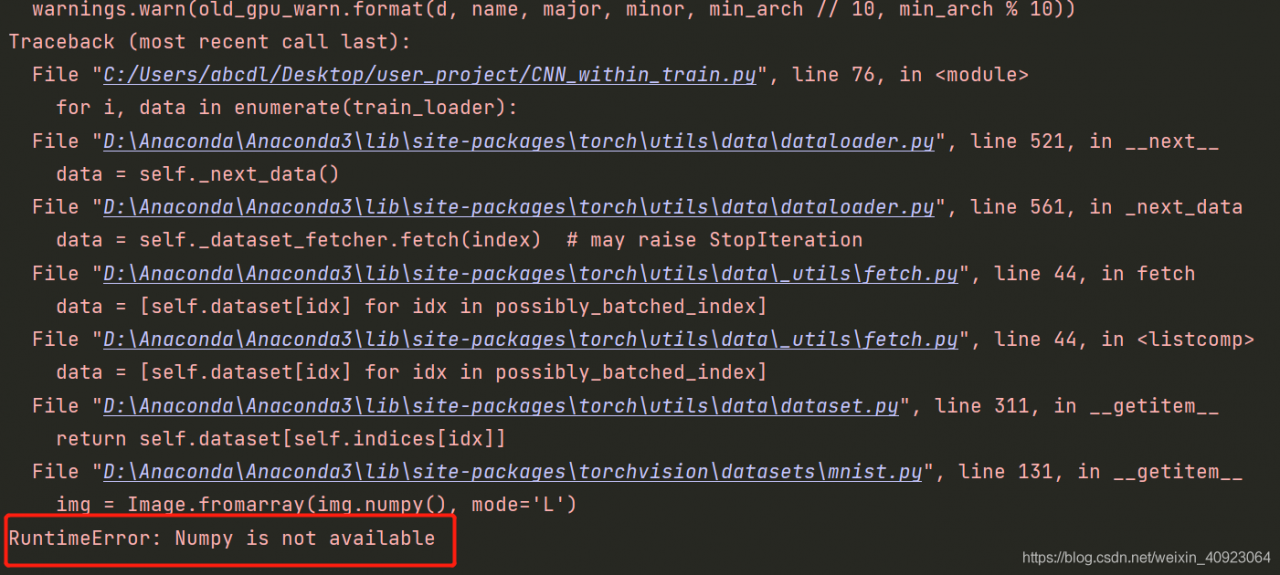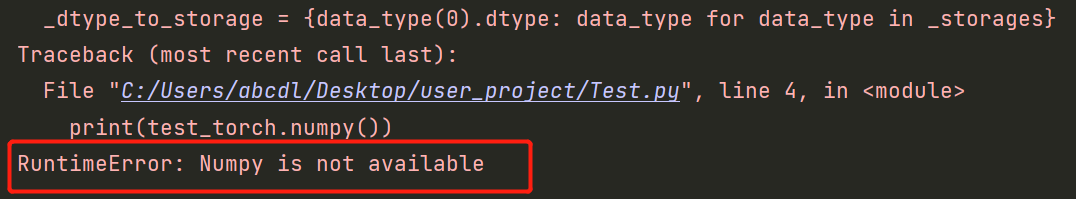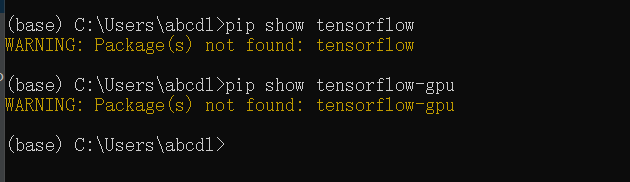# [Solved] RuntimeError: Numpy is not available (Associated Torch or Tensorflow)

Runtimeerror: numpy is not available solution

Problem Description:

Today, the old computer crashed (cried) and the new computer got started. I couldn’t wait to install the pytorch and check the operation of the previous project. As a result, there was an error running the model training``````for i, data in enumerate(train_loader):
``````

The bottom layer of this statement is applied to the operation of converting the tensor variable to numpy, that is

``````import numpy
import torch
test_torch = torch.rand(100,1,28,28)
print(test_torch.numpy())
# will produce the same error
``````Problem root

The direct correlation between pytoch and tensorflow is ignored
if there is only torch but no tensorflow in the environment, the tensor variable used by torch will lose the tensor related operation function, such as torch. Numpy()Solution:

Install tensorflow in the basic environment, whether CPU version or GPU version
in Anaconda environment, you can directly

``````conda install tensorflow
``````

Otherwise pip

``````pip install --ignore-installed --upgrade tensorflow-gpu
``````